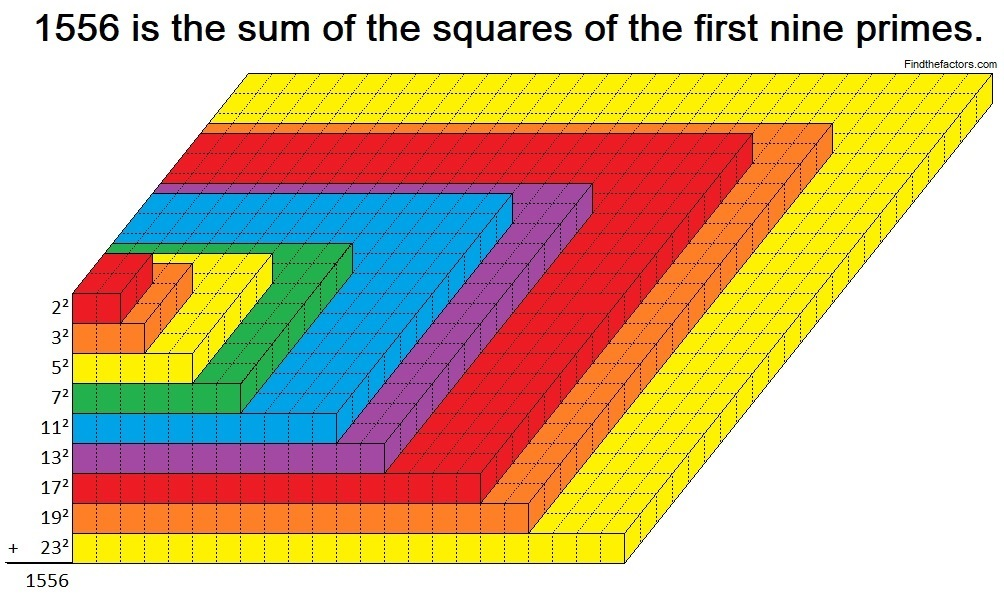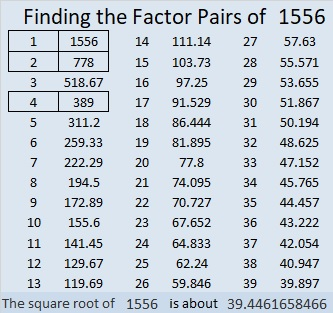# 1556 Stacks Up Nicely!

### What is special about the number 1556?

What makes 1556 stack up?

From OEIS.org we learn that
2² + 3² + 5² + 7² + 11² + 13² + 17² + 19² + 23² =  1556.
Yes, that’s the sum of the squares of the first nine prime numbers.
Those perfect squares can be stacked on top of each other as I illustrate in the graphic below:### Factors of 1556:

1556 (and every other whole number whose last two digits are 56) is divisible by 4:

• 1556 is a composite number.
• Prime factorization: 1556 = 2 × 2 × 389, which can be written 1556 = 2² × 389.
• 1556 has at least one exponent greater than 1 in its prime factorization so √1556 can be simplified. Taking the factor pair from the factor pair table below with the largest square number factor, we get √1556 = (√4)(√389) = 2√389.
• The exponents in the prime factorization are 2 and 1. Adding one to each exponent and multiplying we get (2 + 1)(1 + 1) = 3 × 2 = 6. Therefore 1556 has exactly 6 factors.
• The factors of 1556 are outlined with their factor pair partners in the graphic below.### More about the Number 1556:

1556 is the sum of two squares:
34² + 20² = 1556.

1556 is the hypotenuse of a Pythagorean triple:
756 -1360-1556, which is 4 times (189-340-389).
It can also be calculated from 34² – 20², 2(34)(20), 34² + 20².

1556 is also the difference of two squares:
390²  – 388²  = 1556.